Open in App
Not now

# IBPS Clerk Prelims Reasoning Question Paper 2020

• Last Updated : 25 Mar, 2022

IBPS conducts various banks exams like IBPS Clerk, IBPS PO, IBPS SO etc. to encourage the Recruitment of young talent in various National Public Sector Banks. These exams are conducted to fill 4-5K vacancies every year.

When we talk about the IBPS Clerk exam, it is conducted for the Recruitment of “Clerical Grade” in the various Public sector banks of India. IBPS Clerk Notification 2022 will be released in July 2022,  It will be a golden opportunity for those, who want to serve in Public Sector Banks.

In this article, we have provided “Memory-Based Reasoning Paper of IBPS Clerk Prelims 2020”, to give you an idea of the current pattern of IBPS Clerk and score good marks in the exam.

For more practice read our other articles related to it.

Directions (1-5): Study the following information carefully and answer the question given below.

Eight boxes are placed one above the another. Only one box is placed between box E and C. Box H is placed below box G. Only two boxes are placed between box C and G. Box G is placed below box C. Only two boxes are placed between box F and E.  Three boxes are placed between D and B, both D and B are placed above box E. Not more than one box is placed between box D and box A.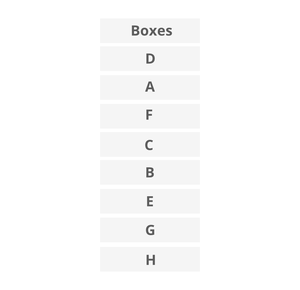Q1. How many boxes are there between box E and box D?

a) None

b) One

c) Two

d) Three

e) More than three

Solution: e

More than three boxes are there between Box E and Box D.

Q2. Which of the following box is placed immediately above box B?

a) H

b) F

c) C

d) E

e) None of these

Solution: c

C box is placed immediately above box B.

Q3. Which of the following box is placed at the bottommost position?

a) H

b) F

c) C

d) E

e) None of these

Solution: a

H box is placed at the bottommost position.

Q4. Which of the following box is placed immediately below box A?

a) H

b) F

c) C

d) E

e) None of these

Solution: b

F box is placed immediately below box A.

Q5. How many boxes are there between box H and box C?

a) None

b) One

c) Two

d) Three

e) More than three

Solution: d

Three boxes are there between box H and box C.

Directions (6-9): In these questions, relationship between different elements is shown in the statements. The statements are followed by conclusions. Give answer

a) If only conclusion I is true

b) If only conclusion II is true

c) If either conclusion I or II is true

d) If neither conclusion I nor II is true

e) If both conclusions I and II are true

Q6. Statements:

B > R ≥ T = H > W ≤ S

Conclusions:

I. H < B

II. S ≥ T

Solution: a

I. H < B(True)

II. S ≥ T(False)

If only conclusion I is true

Q7. Statements:

Y = D ≥ H ≥ S = C ≤ V ≤ B

Conclusions:

I. S < D

II. S = D

Solution: c

I. S < D(False)

II. S = D(False)

If either conclusion I or II is true

Q8. Statements:

J > I ≥ G > F ≤ N < V

Conclusions:

I. J > N

II. V > F

Solution: b

I. J > N(False)

II. V > F(True)

If only conclusion II is true

Q9. Statements:

M < H ≤ D < E > R ≤ Y

Conclusions:

I. E > H

II. M < D

Solution: e

I. E > H(True)

II. M < D(True)

If both conclusions I and II are true

Directions (10-14): Study the following information carefully and answer the question given below

Six athletics are arranged according to their weight in the ascending order from right to left. Y is not the heaviest among all. U is heavier than Z and W. Z is heavier than V and X but not heavier than Y. V and W are not the lightest among all. U is not just heavier than Z. Y is heavier than W, who is not the 3rd heaviest among all. Weight of the 2nd lightest person is 19kg. Total weight of V and W is 46kg. 2nd heaviest person is twice heavy to the one who is 2nd lightest. W is just lighter than Z. Total weight of all the persons are 150kg.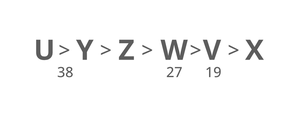Q10. What may be the possible weight of Z?

a) 30kg

b) 39kg

c) 24kg

d) 26kg

e) None of these

Solution: a

30kg possible weight of Z.

Q11. The number of persons is heavier than Z is the same as the number of persons lighter than ___?

a) U

b) W

c) X

d) V

e) None of these

Solution: b

The number of persons is heavier than Z is the same as the number of persons lighter than W.

Q12. If X is 10kg lighter than V and Z is 5kg heavier than W then what is the weight of U?

a) 25kg

b) 43kg

c) 28kg

d) 33kg

e) None of these

Solution: a

25 kg is the weight of U.

Q13. What may be the possible weight of U?

a) 30kg

b) 39kg

c) 38kg

d) 26kg

e) None of these

Solution: b

39kg is the possible weight of U.

Q14. Which of the following statement is true?

a) Only one athletics is lighter to W

b) X is the lightest among all

c) U is lighter to X

d) Weight of U is 50 kg

e) None is true

Solution: b

X is the lightest among all.

Direction (15-18): Study the following arrangement carefully and answer the questions given below:

8 5 2 6 4 5 1 4 9 4 7 3 2 7 2 5 9 3 9 1 8 1 2 5 8 6 3 6 4 2 6 2

Q15. How many 2’s is/are there in the above arrangement, each of which is immediately preceded by a digit that has a numerical value of more than 5?

a) None

b) One

c) Two

d) Three

e) More than three

Solution: c

Two has a numerical value of more than 5.

Q16. Which of the following number is Fifth to the left of the Twenty-one from the left end of the above arrangement?

a) 3

b) 7

c) 2

d) 5

e) 1

Solution: d

5 is fifth to the left of the Twenty-one from the left end of the above arrangement.

Q17. If all the even digits are removed from the above arrangements then, which of the following digit will be Seventh from the right end of the arrangement?

a) 9

b) 5

c) 1

d) 3

e) 7

Solution: a

9 will be seventh from the right end.

Q18. How many such 6’s is there in the above arrangement, each of which is immediately followed by a perfect square?

a) None

b) One

c) Two

d) Three

e) More than three

Solution: c

Two is immediately followed by a perfect square.

Direction (19-23): Study the following information carefully and answer the questions given below: Eight persons S, T, U, V, W, X, Y and Z purchase some products one after another but not necessarily in the same order. Only one-person purchase between T and V. Z purchase just before S. Y purchase before W and after U. At most two persons purchase before V. One-person purchase between Z and T. X purchase just before U.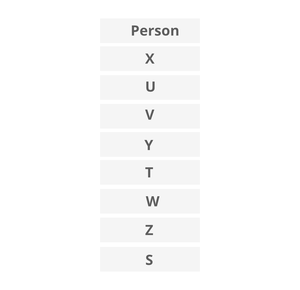Q19. How many persons purchase after T?

a) None

b) Two

c) More than three

d) One

e) None of these

Solution: e

Three persons purchase after T.

Q20. Who among the following purchase just after W?

a) U

b) V

c) Y

d) Z

e) None of these

Solution: d

Z purchase just after W.

Q21. If all the persons are arranged in alphabetical order from top to bottom starting from T, then find how many persons remain at the same position (excluding T)?

a) One

b) None

c) Two

d) Four

e) More than Four

Solution: d

Four persons remain in the same position.

Q22. Who among the following purchase exactly between T and V?

a) U

b) Y

c) X

d) S

e) None of these

Solution: b

Y purchase exactly between T and V.

Q23. How many persons purchase between U and Z?

a) Five

b) Four

c) Three

d) None

e) Two

Solution: b

Four persons purchase between U and Z.

Q24. How many pairs of letters are there in the word “SINGAPORE” which has as many letters between them as we have in the English alphabetical series (from both forward and backward direction)?

a) Three

b) One

c) Two

d) None

e) More than three

Solution: e

Four pairs of letters are there in the word.

Directions (25-28): In each question below are given some statements followed by two conclusions numbered I and II. You have to take the given statements to be true even if they seem to be at variance with commonly known facts and then decide which of the given conclusions logically follows from the given statements, disregarding commonly known facts. Give answer:

Statements:

All F is B.

No C is S.

Only a few F are C.

Conclusions:

I. All F can be S.

II. All S Being F is a possibility.

a) If only conclusion I follows.

b) If only conclusion II follows.

c) If either conclusion I or II follows.

d) If neither conclusion I nor II follows.

e) If both conclusions I and II follow.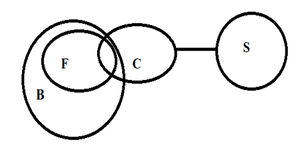Solution: b

If only conclusion II follows.

Q26. Statements

All North are West.

No East is a North.

Some West are middle.

Conclusions:

I. All East are West is a possibility.

II. All West are Middle is a possibility.

a) If only conclusion I follows.

b) If only conclusion II follows.

c) If either conclusion I or II follows.

d) If neither conclusion I nor II follows.

e) If both conclusions I and II follow.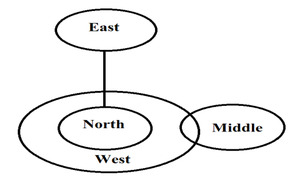Solution: e

If both conclusions I and II follow.

Q27. Statements:

Few Chair are Pen.

Some Book are not Chair.

Every Paper is Book.

Conclusions:

I. Some Paper being pen  is a possibility.

II. All Book Can be Chair.

a) If only conclusion I follows.

b) If only conclusion II follows.

c) If either conclusion I or II follows.

d) If neither conclusion I nor II follows.

e) If both conclusions I and II follow.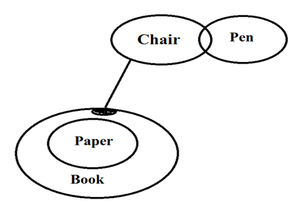Solution: a

If only conclusion I follows.

Q28. Statements:

All chair are bat.

Only a few fan are chair.

Maximum table is bat.

Conclusions:

I. Some fan are bat.

II. All fan can be table.

a) If only conclusion I follows.

b) If only conclusion II follows.

c) If either conclusion I or II follows.

d) If neither conclusion I nor II follows.

e) If both conclusions I and II follow.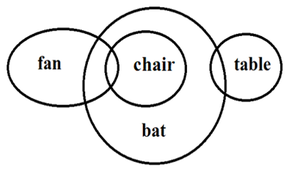Solution: e

If both conclusions I and II follow.

Directions (29-33): Study the following information carefully and answer the question given below Eight persons M, N, O, P, Q, R, S and T were born in the month of October of 4 different years i.e. 1966, 1967, 1968 and 1969 either on 16th or 25th but not necessarily in the same order. T was born just after P. Q was born in the even numbered year but not the eldest among all. More than two persons were born between Q and P. There are as many persons born before T as same as born after N. S was born before R and after M and they all were born on the same date.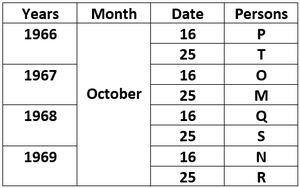Q29. How many persons born before O?

a) One

b) None

c) Two

d) More than four

e) Four

Solution: c

Two persons born before O.

Q30. In which of the following year N was born?

a) 1966

b) 1969

c) Either 1966 or 1969

d) 1967

e) 1968

Solution: b

N was born 1969.

Q31. How many persons were born between M and R?

a) Three

b) Five

c) Two

d) One

e) None of these

Solution: a

Three persons were born between M and R.

Q32. Four of the following five are alike in a certain way and so form a group. Find the one who does not belong to that group?

a) P

b) N

c) Q

d) O

e) T

Solution: e

T does not belong to that group.

Q33. The number of persons born between S and T is same as between ___ and ___?

a) Q, R

b) N, T

c) O, N

d) P, S

e) None of these

Solution: c

The number of persons born between S and T is same as between O and N.

Direction(34-35): Answer the questions based on the information given below:

Point K is 8m north of point I, which is 4m east of point J. Point J is the midpoint of point G and point F. Point K is midpoint of point L and point H. Point H is 6m north of point F. Point L is east of point K. Point G is either north or south of J.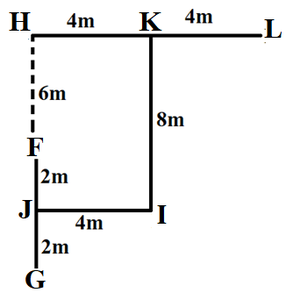Q34. What is the direction of point G with respect to the point I?

a) Northwest

b) Northeast

c) Southwest

d) Southeast

e) None of these

Solution: c

Southwest direction of G respect to T.

Q35. What is the shortest distance between F and L?

a) 8m

b) 10m

c) 9m

d) 15m

e) None of these

Solution: b

10m shortest distance between F and L.

My Personal Notes arrow_drop_up